# Julia-Wolff-Carathéodory theorem

(diff) ← Older revision | Latest revision (diff) | Newer revision → (diff)

Julia–Carathéodory theorem, Julia–Wolff theorem

A classical statement which combines the celebrated Julia theorem from 1920 [a18], Carathéodory's contribution from 1929 [a7] (see also [a8]), and Wolff's boundary version of the Schwarz lemma from 1926 [a30].

Letbe the open unit disc in the complex plane, and let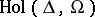be the set of all holomorphic functions onwith values in a domainin(cf. also Analytic function). For the set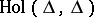, of holomorphic self-mappings on, one writes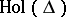; it is a semi-group of holomorphic mappings with respect to composition.

Foron the unit circle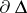, the boundary of, and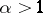, a non-tangential approach region atis defined by(a1)

The term "non-tangential" refers to the fact that at the point,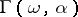lies in the sector(in) that is the region between two straight lines inmeeting atand symmetric about the radius to, the boundary curves ofhaving a corner at, with angle less than.

A function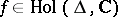is said to have a non-tangential limitatifexists in each non-tangential region. In this case one also writes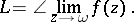Forand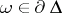, let(a2)

and for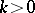, let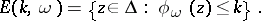(a3)

The setis a closed disc internally tangent to the circle atwith centreand radius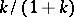. Such a disc is called a horodisc (cf. also Horosphere).

In 1920, G. Julia [a18] identified hypotheses showing how to get the existence of the non-tangential limit at a given boundary point.

## Julia's lemma.

Letbe not constant. Suppose that there are pointsandon the boundary, such that for a sequence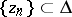converging tothe sequenceconverges toand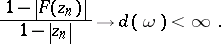(a4)

Then

i)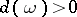;

ii), i.e.for all;

iii)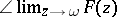exists and is equal to. Moreover, if the equality in ii) holds for some, thenis an automorphism of the disc.

## Julia–Carathéodory theorem.

In 1929, C. Carathéodory [a7] proved that under Julia's hypotheses the derivative also admits a non-tangential limit at the same boundary point.

Suppose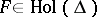. Then the following statements are equivalent:

i)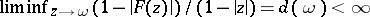, where the limit is taken asapproachesunrestrictedly in;

ii)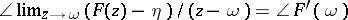exists for some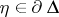;

iii)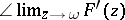exists, and. Moreover,

a)in i);

b) the boundary pointsin ii) and iii) are the same;

c).

After appropriate preliminary rotations, one may assume that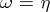. Thus, these results show that ifhas an angular derivative at some boundary pointsuch that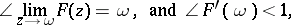thencannot have an interior fixed point in.

Now assume only thathas no interior fixed point in. The question then is: Does the angular derivative at a certain point on the boundary exist?

The affirmative answer was given by J. Wolff [a30] in 1926.

## Wolff's theorem.

Supposehas no fixed point in. Then there is a unique unimodular pointsuch that

i);

ii)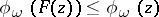;

iii)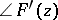exists and is less than or equal to.

The latter assertion can be interpreted as a direct analogue of the Schwarz–Pick lemma (cf. Schwarz lemma), where the role of the fixed point is taken over by a point on the unit circle. Moreover, this result is the key to all the deeper facts about sequences of iterates.

## Generalizations.

There are various versions and proofs of the Julia–Carathéodory theorem (sometimes also called the Julia–Wolff–Carathéodory theorem or Julia–Wolff theorem). For the one-dimensional case, see, for example, [a19], [a29], [a27], [a12], [a26], [a6], [a21] or [a8], [a22], [a28], [a9].

Note that D. Sarason [a26] gave an interesting proof of the Julia–Carathéodory theorem by using Hilbert space constructions for angular derivatives.

A strengthened version of Julia's lemma was established by P.R. Mercer [a21], employing techniques for the hyperbolic Poincaré metric (cf. Poincaré model).

Different generalizations of the Julia–Wolff–Carathéodory theorem for bounded domains inare known: for the unit ball in([a16], [a25]), for the poly-disc ([a17], [a4]), for strongly convex and strongly pseudo-convex domains ([a2], [a3]). Also, M. Abate and R. Tauraso [a1] have described a general framework allowing one to generalize the Julia–Wolff–Carathéodory theorem in terms of the Kobayashi metric (cf. also Hyperbolic metric; Kobayashi hyperbolicity) on a bounded domain in.

For generalizations of Wolff's theorem in the unit ball of a complex Hilbert space, see [a13] and [a14].

Earlier, V.P. Potapov [a23] extended Julia's lemma to matrix-valued holomorphic mappings of a complex variable. His results, as well as the Julia–Wolff–Carathéodory theorem, were generalized by K. Fan and T. Ando ([a10], [a11] and [a5]) to operator-valued holomorphic mappings. Also, in these works they extended the Julia–Wolff–Carathéodory theorem to holomorphic mappings of proper contractions on the unit Hilbert ball acting in the sense of functional calculus.

K. Wlodarczyk [a31] and P. Mellon [a20] have presented some more general results in this direction for the holomorphic mappings on the open unit ball of so-called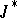-algebras, using techniques developed by L.A. Harris [a15].

For a survey of work in higher dimensions, see [a25], [a14], [a9], [a24], [a20], [a1].

How to Cite This Entry:
Julia-Wolff-Carathéodory theorem. Encyclopedia of Mathematics. URL: http://encyclopediaofmath.org/index.php?title=Julia-Wolff-Carath%C3%A9odory_theorem&oldid=12290
This article was adapted from an original article by David Shoikhet (originator), which appeared in Encyclopedia of Mathematics - ISBN 1402006098. See original article